Simplex (abstract)

A topological spacewhose points are non-negative functionson a finite setsatisfying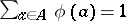. The topology on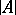is induced from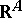, the space of all functions frominto. The real numbers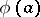are called the barycentric coordinates of the point, and the dimension ofis defined as. In caseis a linearly independent subset of a Euclidean space,is homeomorphic to the convex hull of the set(the homeomorphism being given by the correspondence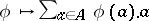). The convex hull of a linearly independent subset of a Euclidean space is called a Euclidean simplex.

For any mappingof finite sets, the formula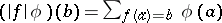,, defines a continuous mapping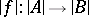, which, for Euclidean simplices, is an affine (non-homogeneous linear) mapping extending. This defines a functor from the category of finite sets into the category of topological spaces. Ifandis the corresponding inclusion mapping, then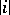is a homeomorphism onto a closed subset of, called a face, which is usually identified with. Zero-dimensional faces are called vertices (as a rule, they are identified with the elements of).

A topological ordered simplex is a topological spacetogether with a given homeomorphism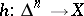, where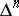is a standard simplex. The images of the faces ofunderare called the faces of the topological ordered simplex. A mappingof two topological ordered simplicesandis said to be linear if it has the form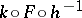, whereandare the given homeomorphisms andis a mapping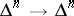of the form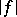.

A topological simplex (of dimension) is a topological spaceequipped withhomeomorphisms(that is, with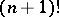structures of a topological ordered simplex) that differ by homeomorphismsof the form, whereis an arbitrary permutation of the vertices. Similarly, a mapping of topological simplices is called linear if it is a linear mapping of the corresponding topological ordered simplices.

Elements of simplicial sets (cf. Simplicial set) and distinguished subsets of simplicial schemes (cf. Simplicial scheme) are also referred to as simplices.